# Images of the Experiment

## Others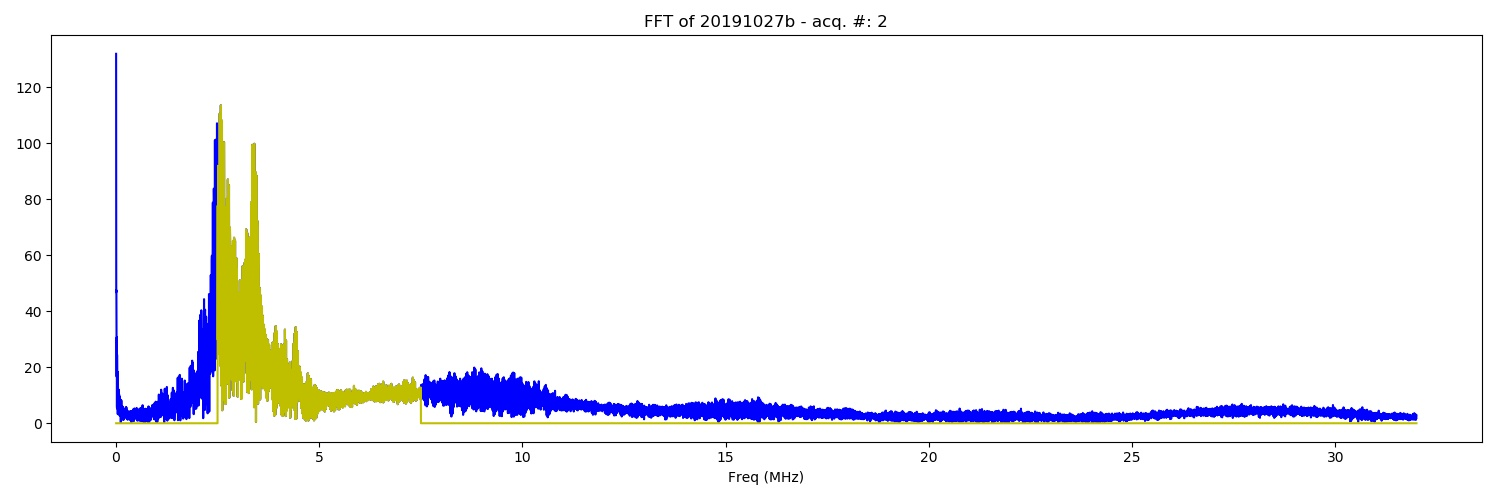FFT of the of 20191027b experiment. De.bin DAC (100) from 100 to 900, True - DAC = 400 (category: FFT).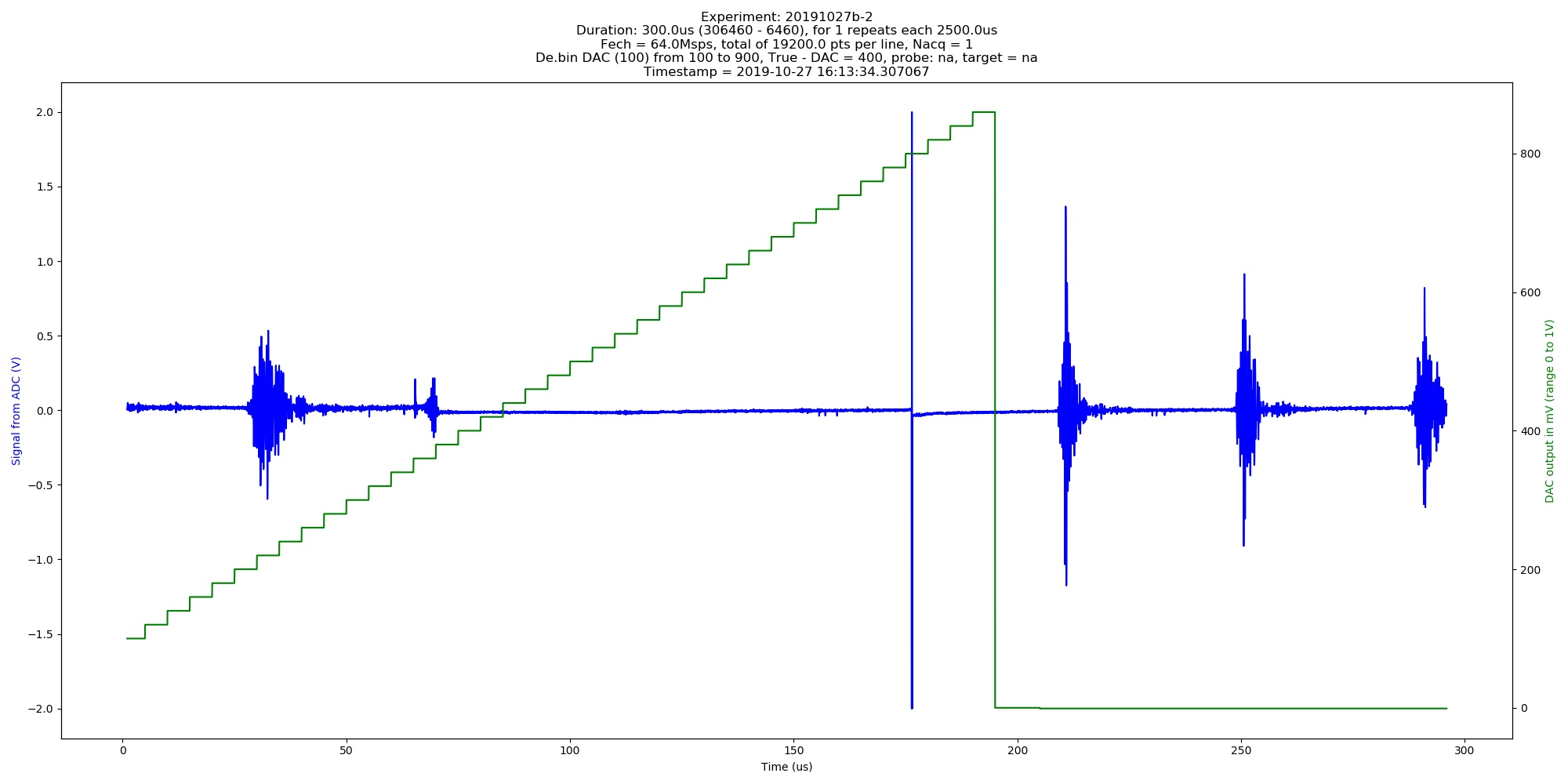Automated image of 20191027b experiment. De.bin DAC (100) from 100 to 900, True - DAC = 400 (category: graph).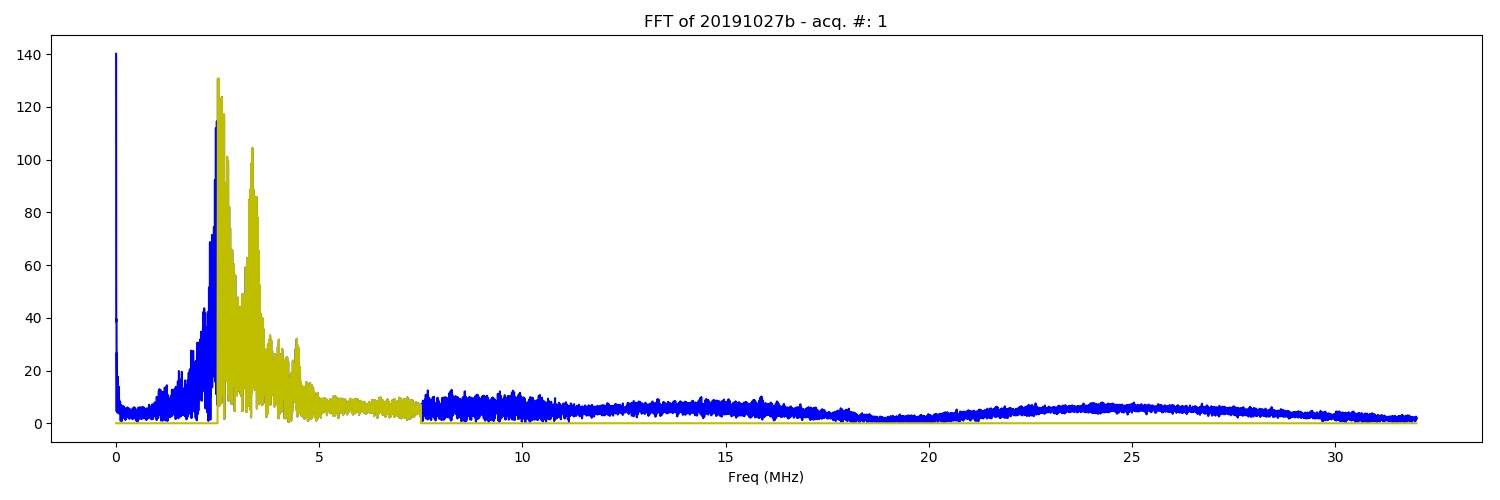FFT of the of 20191027b experiment. De.bin DAC (100) from 100 to 900, True - DAC = 400 (category: FFT).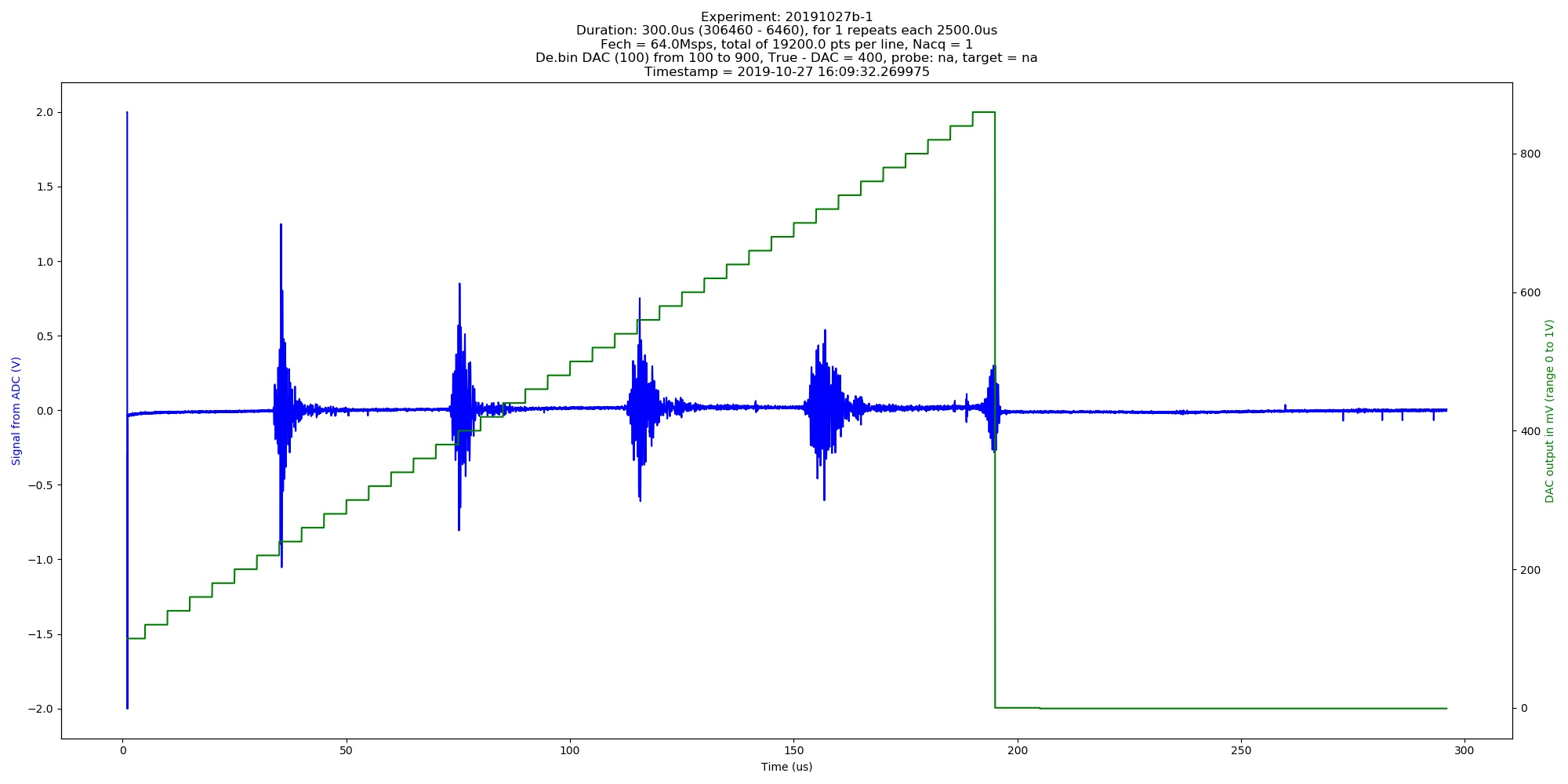Automated image of 20191027b experiment. De.bin DAC (100) from 100 to 900, True - DAC = 400 (category: graph).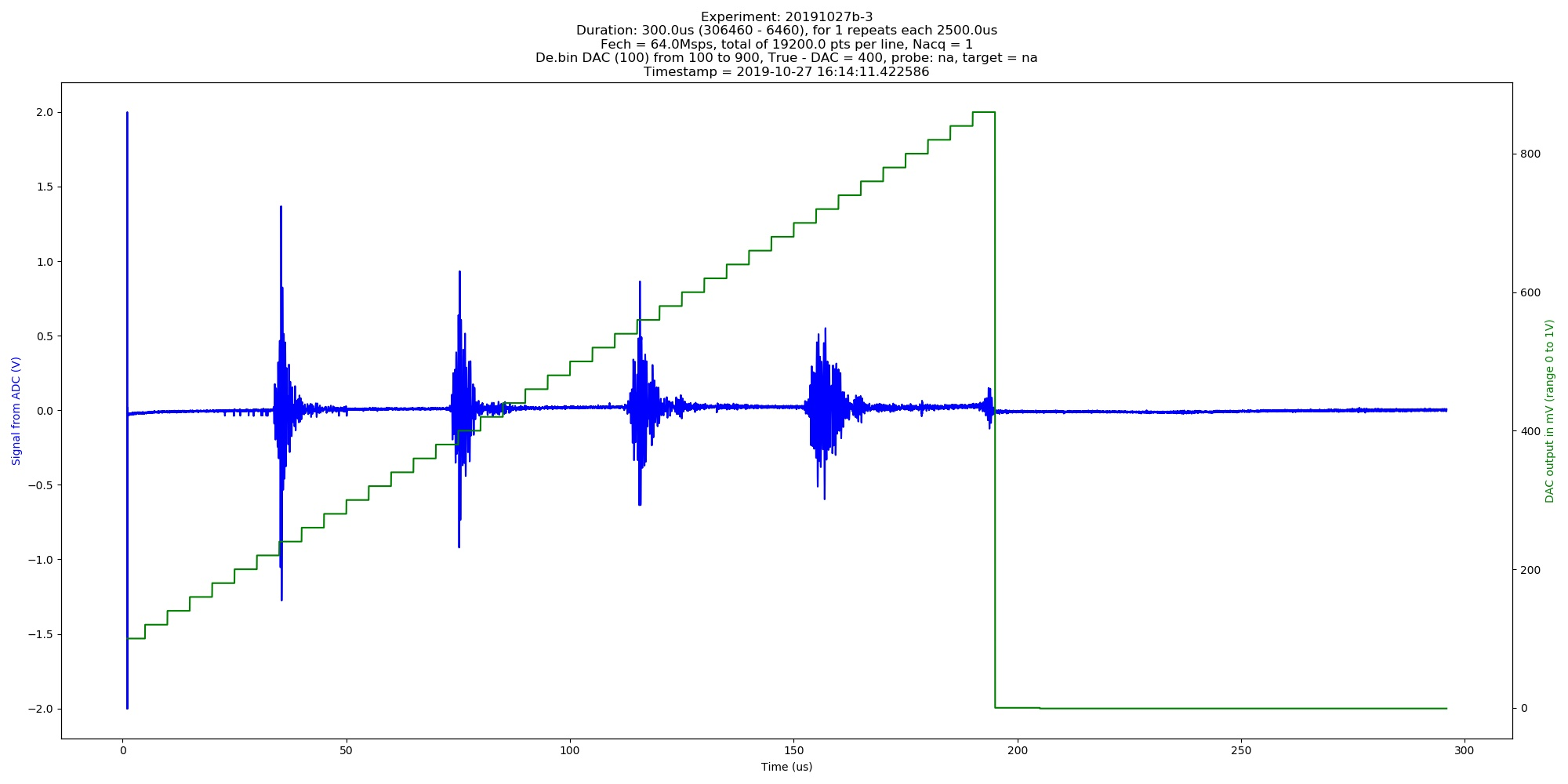Automated image of 20191027b experiment. De.bin DAC (100) from 100 to 900, True - DAC = 400 (category: graph).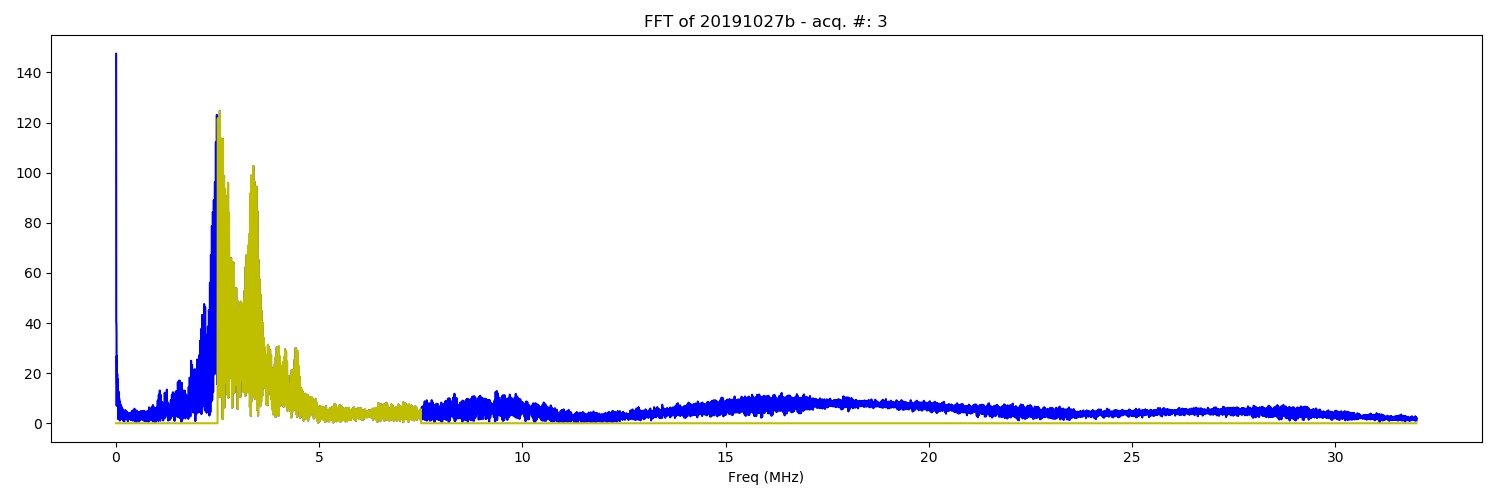FFT of the of 20191027b experiment. De.bin DAC (100) from 100 to 900, True - DAC = 400 (category: FFT).